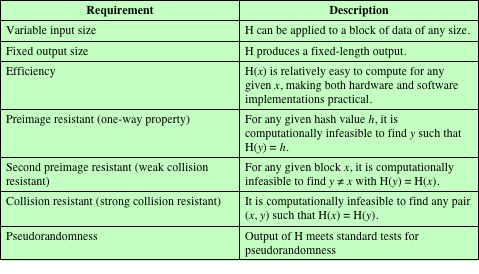# List out criteria of a cryptographic hash function ?

• A hash function H accepts a variable-length block of data M as input and produces a fixed-size hash value as output
• h = H(M)
• Principal objective of hash function  is data integrity - A change to any bit or bits in M results, in a change to the hash code.
• Cryptographic hash function
• The kind of hash function needed for security applications is referred to as a cryptographic hash function
• The algorithm for this should be computationally infeasible to find either:

(a) a data object that maps to a pre-specified hash result (the one-way property)

(b) two data objects that map to the same hash result (the collision-free property)

Applications of Cryptographic Hash Functions

• Main Applications are in
1.  Message authentication and
2.  Digital signatures
• Other applications are:
• to create a one-way password file
• intrusion detection and virus detection
• to construct a pseudorandom number generator (PRNG).

Security Requirements for a Cryptographic Hash Function H